Select Page

In the previous article, we started exploring some of the basic machine learning algorithms and learned how to use ML.NET. There we covered Linear Regression, its variations and we implemented it from scratch with C#. In this article, we focus on the classification algorithm or to be more precise, the algorithms that are used primarily for classification problems. Note that we will not cover all the classification algorithms, for example, SVM and Decisions Trees, because these algorithms can be used for regression as well, so they will get separated articles just for them.

Are you afraid that AI might take your job? Make sure you are the one who is building it.

STAY RELEVANT IN THE RISING AI INDUSTRY! 🖖

1. Dataset and Prerequisites
2. Understanding classification algorithms
3. ML.NET Classification Algorithms
4. Binary Classification with ML.NET
5. Multiclass Classification with ML.NET

## 1. Dataset and Prerequisites

Data that we use in this article is from PalmerPenguins Dataset. This dataset has been recently introduced as an alternative to the famous Iris dataset. It is created by Dr. Kristen Gorman and the Palmer Station, Antarctica LTER. You can obtain this dataset here, or via Kaggle. This dataset is essentially composed of two datasets, each containing data of 344 penguins. Just like in Iris dataset there are 3 different species of penguins coming from 3 islands in the Palmer Archipelago. Also, these datasets contain culmen dimensions for each species. The culmen is the upper ridge of a bird’s bill. In the simplified penguin’s data, culmen length and depth are renamed as variables culmen_length_mm and culmen_depth_mm.Here is what that data looks like: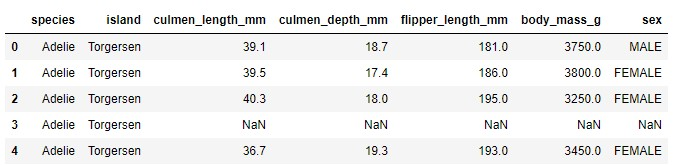The implementations provided here are done in C#, and we use the latest .NET 5. So make sure that you have installed this SDK. If you are using Visual Studio this comes with version 16.8.3. Also, make sure that you have installed the following package:

``Install-Package Microsoft.ML``

You can do a similar thing using Visual Studio’s Manage NuGetPackage option: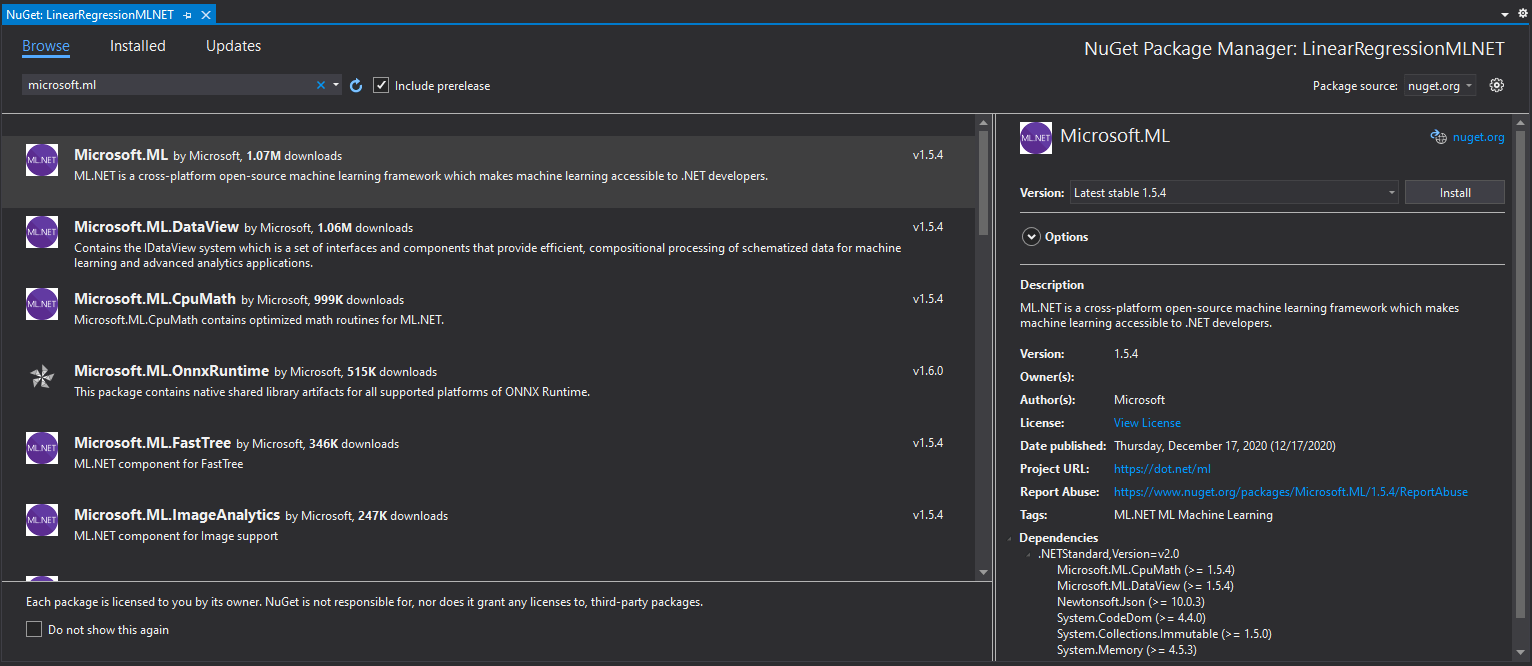If you need to catch up with the basics of machine learning with ML.NET check out this article. Apart from that, you should be comfortable with the basics of linear algebra.

## 2. Understanding Classification Algorithms

When we are solving classification problems we want to predict the class label of the observed sample. For example, we want to predict a class of penguins based on their bill length and width. There are several approaches to solving this. As we will see some of the solutions are based on calculating distances, while others are based on creating a probabilistic model. One way or another, the goal is to create function y = f(X) that minimizes the error of misclassification, where X is the set of observations and y is the output class label.

### 1.1 Logistic Regression

The first algorithm that we explore in this article is Logistic Regression. The name might be a bit confusing because it comes from statistics and it is due to the similar mathematical formulation for Linear Regression. Just so simplify things even more for this first algorithm, we explain it in the case of binary classification, meaning we have only two classes. As we mentioned, this algorithm has a similar formulation as linear regression. What we want to do is to model yi as a linear function of xi, but that is not as simple now when yi can have only two values (two classes remember). So, the Logistic Regression model still computes a weighted sum of the input features and adds a bias term, but instead of outputting the result directly, it does some extra processing.

That is why we assign value 0 to the first class (negative class) and value 1 to the second class (positive class). That is how the problem is transformed into the problem of finding a continuous function whose codomain is (0, 1). This means that we want to estimate the probability that an observed sample belongs to a particular class. For that purpose, sigmoid function or standard logistic function is used: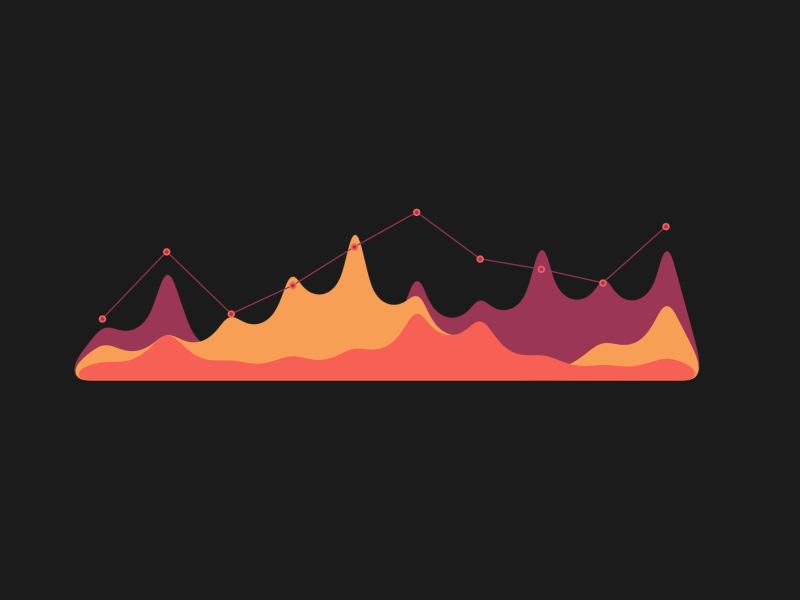So, with Logistic Regression we still calculate wX + b (or to simplify it even further and put all parameters into the matrix – θX) value and put the result in sigmoid function. If the result is greater than 0.5 (probability is larger than 50%), then the model predicts that the instance belongs to that class positive class(1), or else it predicts that it does not belong to it (negative class). Mathematically we can put it like this: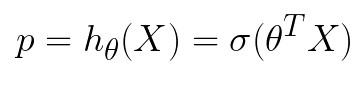It is important to note that we need to modify loss function as well in order for it to work on this type of data. For this purpose we use log loss function which is defined like this: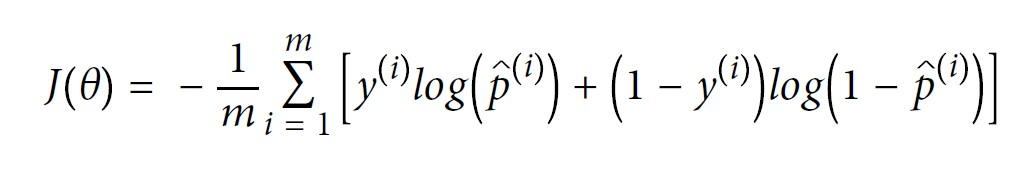Unlike the loss function that we used for the Linear Regression, this formula doesn’t have its closed form. We can not use the Normal Equation, so we need to use gradient descent to optimize it. For that purpose we need to calculate partial derivatives of the cost function with regards to the jth model parameter θj: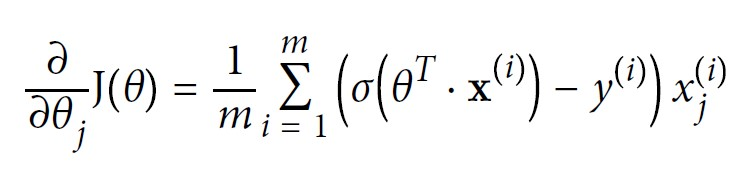Ok, that would be rough theory behind it, let’s move to the implementation.### 2.2 K-Nearest Neighbours (KNN)

Unlike Logistic Regression, this algorithm is not calculating probabilities but is based on distances. This effectively means that it is non-parametric models, but it also means that it keeps all training data in memory after the training. In fact, storing training data is the training process. Basically, once a new previously unseen sample is passed into the algorithm it calculates k training examples that are closest to x and returns the majority label (or average label, depending on the implementation). The distances can be calculated in various different ways. Euclidean distance or Cosine similarity are often used in practice, but you can play around with Manhattan distance or Chebychev distance. In this article we use Euclidean distance which can be described with the formula:To sum it up, this algorithm is simple and intuitive and it can be broken down into several steps:

• Decide the number of neighbors that algorithm considers
• Store training data with corresponding labels in memory
• Once a new input point comes in, calculate it’s the distance from the training points based on the distance function of your choosing
• Sort the results and pick k points that are closest to the new input sample
• Detect the label of the majority of k points and assign this label to new input sample### 2.3 Naive Bayes

Third and final algorithm we explore today is the Naive Bayes algorithm. As we mentioned previously, classification problems can be solved by creating a predictive model. That is what we have done with Logistic Regression. Another way to create a predictive model would be to estimate the conditional probability of the class label, given the observation. Meaning, we can calculate conditional probability for each class label in and the pick the label with the highest probability as most likley label. In theory, Bayes Theorem can be used for this: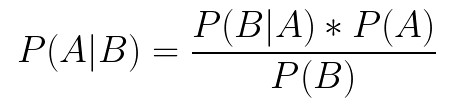The main problem with this approach is that we need a really large dataset to calculate the conditional probability P(x1, x2, …, xn | yi), because this formula assumes that each input variable is dependent upon all other variables. If the number of features is large, the size of the dataset becomes an even bigger problem. To simplify this problem we assume that each input variable as being independent of each other. This might sound weird…because it is 🙂 In reality, it is really rare that input features don’t depend on each other. However, this approach proved to surprisingly well in the wild. That is why we can rewrite the formula from above as:To calculate P(yi) all we have to do is divide the frequency of class yi in the training dataset and divide it with the total number of samples in the training set (P(yi) = # of samples with yi / total # of examples). The second part of the equation, the conditional probability, can be derived from data as well. So, let’s implement it.## 3. ML.NET Classification Algorithms

In general, ML.NET provides two sets of algorithms for classification – Binary classification algorithms and Multiclass classification algorithms. As the name suggests, the first ones are doing simple classification of two classes, meaning it is able to detect if some data belongs to some class or not. Multiclass classification algorithms are able to distinguish between multiple classes.

Binary classification algorithms supported in ML.NET are:

• LBFGS Logistic Regression – it is a variation of the Logistic Regression that is based on the limited memory Broyden-Fletcher-Goldfarb-Shanno method (L-BFGS).
• Prior –  Uses prior distribution for 0/1 class labels and outputs that
• SDCA Logistic Regression – it is a variation of logistic regression that is based on the Stochastic Dual Coordinate Ascent (SDCA) method. The algorithm can be scaled because it’s a streaming training algorithm as described in a KDD best paper.
• SDCA Non-Calibrated – The version of the previous algorithm that is not calibrated.
• SGD Calibrated – it is a variation of logistic regression that is based on the Stochastic Gradient Descent.
• SGD Non-Calibrated – The version of the previous algorithm that is not calibrated.Multi-class classification algorithms supported in ML.NET are:

• LBFGS Maximum Entropy – The major difference between the maximum entropy model and logistic regression is the number of classes supported. Logistic regression is used for binary classification while the maximum entropy model handles multiple classes.  This one uses is based on the limited memory Broyden-Fletcher-Goldfarb-Shanno method (L-BFGS).
• Naive Bayes
• One Versus All – This is an interesting algorithm that performs a binary classification algorithm for each class of the dataset and creating multiple binary classifiers. Prediction is then performed by running these binary classifiers and choosing the prediction with the highest confidence score.
• SDCA Maximum Entropy – It is maximum entropy algorithms (logistic regression generalization) based on the Stochastic Dual Coordinate Ascent (SDCA) method.
• SDCA Non-Calibrated – The version of the previous algorithm that is not calibrated.

## 4. Binary Classification with ML.NET

As the name suggests, Binary classification is performing simple classification on two classes. In essence, it is used for detecting if some sample represented some event or not. So, simple true-false predictions. That is why we had to modify and pre-process data from PalmerPenguin Dataset. We left two features culmen depth and culmen length. The other features are removed. We also modified the species feature, which now indicated if the sample belongs to the Adelie species or not (1 if the sample represents Adelie; 0 otherwise). Here is how data looks like now:This is a simplified dataset and the problem we want to learn – Does some new sample that comes in our system represents Adelie’s class or not. Let’s see how we can do that with ML.NET.

### 4.1 High-Level Architecutre

Before we dive into the ML.NET implementation, let’s consider the high-level architecture of this implementation. In general, we want to build an easily extendable solution that we can easily extend with new binary classification algorithms that ML.NET might include in the future. That is why the folder structure of our solution looks like this: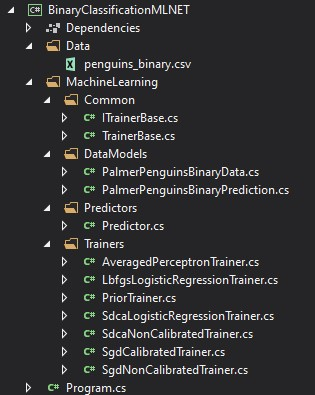The Data folder contains .csv with input data and the MachineLearning folder contains everything that is necessary for our algorithm to work. The architectural overview can be represented like this: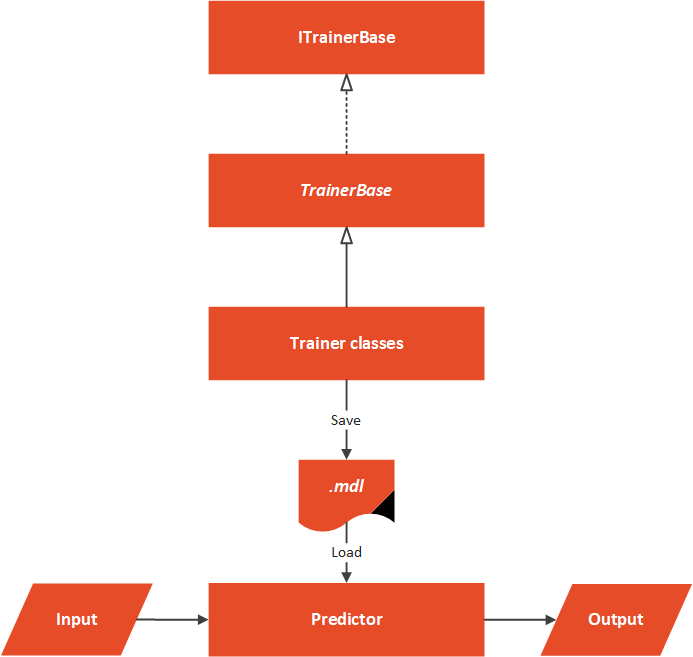At the core of this solution, we have an abstract TrainerBase class. This class is in the Common folder and its main goal is to standardize the way this whole process is done. It is in this class where we process data and perform feature engineering. This class is also in charge of training machine learning algorithm. The classes that implement this abstract class are located in the Trainers folder. Here we can find multiple classes which utilize ML.NET algorithms. These classes define which algorithm should be used. In this particular case, we have only one Predictor located in the Predictor folder.

### 4.2 Data Models

In order to load data from the dataset and use it with ML.NET algorithms, we need to implement classes that are going to model this data. Two files can be found in Data Folder: PalmerPenguinBinaryData and PricePalmerPenguinBinaryPredictions. The PalmerPenguinBinaryData class models input data and it looks like this:

``````using Microsoft.ML.Data;

namespace BinaryClassificationMLNET.MachineLearning.DataModels
{
/// <summary>
/// Models Palmer Penguins Binary Data.
/// </summary>
public class PalmerPenguinsBinaryData
{
public bool Label { get; set; }

public float CulmenLength { get; set; }

public float CulmenDepth { get; set; }
}
}
``````The PricePalmerPenguinBinaryPredictions class models output data:

``````using Microsoft.ML.Data;

namespace BinaryClassificationMLNET.MachineLearning.DataModels
{
/// <summary>
/// Models Palmer Penguins Binary Prediction.
/// </summary>
public class PalmerPenguinsBinaryPrediction
{
public bool PredictedLabel { get; set; }
}
}
``````

### 4.3 TrainerBase and ITrainerBase

As we mentioned, this class is the core of this implementation. In essence, there are two parts to it. The first one is the interface that describes this class and another is the abstract class that needs to be overridden with the concrete implementations, however, it implements interface methods. Here is the ITrainerBase interface:

``````using Microsoft.ML.Data;

namespace BinaryClassificationMLNET.MachineLearning.Common
{
public interface ITrainerBase
{
string Name { get; }
void Fit(string trainingFileName);
BinaryClassificationMetrics Evaluate();
void Save();
}
}``````

The TrainerBase class implements this interface. However, it is abstract since we want to inject specific algorithms:

``````using BinaryClassificationMLNET.MachineLearning.DataModels;
using Microsoft.ML;
using Microsoft.ML.Calibrators;
using Microsoft.ML.Data;
using Microsoft.ML.Trainers;
using Microsoft.ML.Transforms;
using System;
using System.IO;

namespace BinaryClassificationMLNET.MachineLearning.Common

{
/// <summary>
/// Base class for Trainers.
/// This class exposes methods for training, evaluating and saving ML Models.
/// Classes that inherit this class need to assing concrete model and name; and to implement data pre-processing.
/// </summary>
public abstract class TrainerBase<TParameters> : ITrainerBase
where TParameters : class
{
public string Name { get; protected set; }

protected static string ModelPath => Path
.Combine(AppContext.BaseDirectory, "classification.mdl");

protected DataOperationsCatalog.TrainTestData _dataSplit;
protected ITrainerEstimator<BinaryPredictionTransformer<TParameters>, TParameters> _model;
protected ITransformer _trainedModel;

protected TrainerBase()
{
MlContext = new MLContext(111);
}

/// <summary>
/// Train model on defined data.
/// </summary>
/// <param name="trainingFileName"></param>
public void Fit(string trainingFileName)
{
if (!File.Exists(trainingFileName))
{
throw new FileNotFoundException(\$"File {trainingFileName} doesn't exist.");
}

var dataProcessPipeline = BuildDataProcessingPipeline();
var trainingPipeline = dataProcessPipeline.Append(_model);

_trainedModel = trainingPipeline.Fit(_dataSplit.TrainSet);
}

/// <summary>
/// Evaluate trained model.
/// </summary>
/// <returns>Model performance.</returns>
public BinaryClassificationMetrics Evaluate()
{
var testSetTransform = _trainedModel.Transform(_dataSplit.TestSet);

return MlContext.BinaryClassification.EvaluateNonCalibrated(testSetTransform);
}

/// <summary>
/// Save Model in the file.
/// </summary>
public void Save()
{
MlContext.Model.Save(_trainedModel, _dataSplit.TrainSet.Schema, ModelPath);
}

/// <summary>
/// Feature engeneering and data pre-processing.
/// </summary>
/// <returns>Data Processing Pipeline.</returns>
private EstimatorChain<NormalizingTransformer> BuildDataProcessingPipeline()
{
var dataProcessPipeline = MlContext.Transforms.Concatenate("Features",
nameof(PalmerPenguinsBinaryData.CulmenDepth),
nameof(PalmerPenguinsBinaryData.CulmenLength)
)
.Append(MlContext.Transforms.NormalizeMinMax("Features", "Features"))
.AppendCacheCheckpoint(MlContext);

return dataProcessPipeline;
}

{
var trainingDataView = MlContext.Data
return MlContext.Data.TrainTestSplit(trainingDataView, testFraction: 0.3);
}
}
}
``````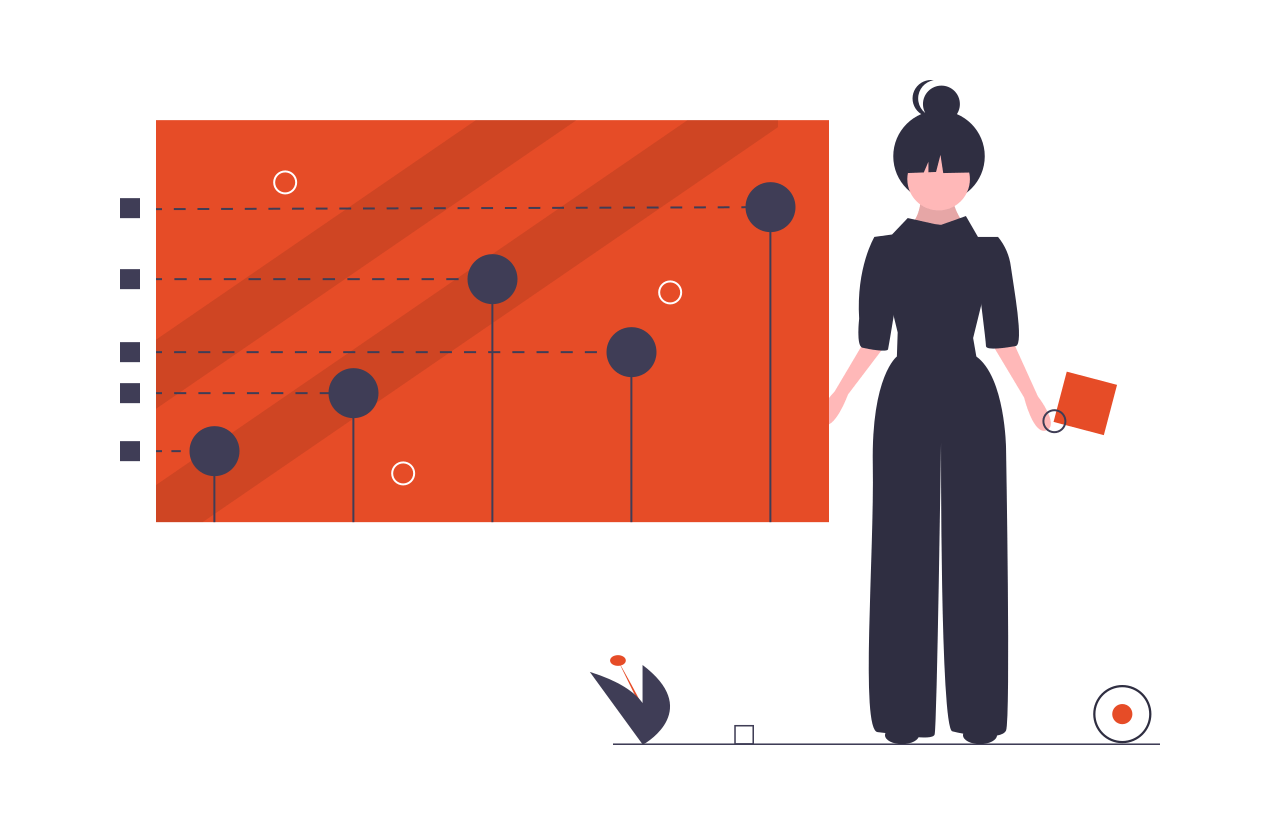That is one large class. It controls the whole process. Let’s split it up and see what it is all about. First, let’s observe the fields and properties of this class:

``````public string Name { get; protected set; }

protected static string ModelPath => Path.Combine(AppContext.BaseDirectory, "classification.mdl");

protected DataOperationsCatalog.TrainTestData _dataSplit;
protected ITrainerEstimator<BinaryPredictionTransformer<TParameters>, TParameters> _model;
protected ITransformer _trainedModel;``````

The Name property is used by the class that inherits this one to add the name of the algorithm. The ModelPath field is there to define where we will store our model once it is trained. Note that the file name has .mdl extension. Then we have our MlContext so we can use ML.NET functionalities. Don’t forget that this class is a singleton, so there will be only one in our solution. The _dataSplit field contains loaded data. Data is split into train and test datasets within this structure.

The field _model is used by the child classes. These classes define which machine learning algorithm is used in this field. The _trainedModel field is the resulting model that should be evaluated and saved. In essence, the only job of the class that inherits and implements this one is to define the algorithm that should be used, by instantiating an object of the desired algorithm as _model

Cool, let’s now explore Fit() method:

``````public void Fit(string trainingFileName)
{
if (!File.Exists(trainingFileName))
{
throw new FileNotFoundException(\$"File {trainingFileName} doesn't exist.");
}

var dataProcessPipeline = BuildDataProcessingPipeline();
var trainingPipeline = dataProcessPipeline.Append(_model);

_trainedModel = trainingPipeline.Fit(_dataSplit.TrainSet);
}``````

This method is the blueprint for the training of the algorithms. As an input parameter, it receives the path to the .csv file. After we confirm that the file exists we use the private method LoadAndPrepareData. This method loads data into memory and splits it into two datasets, train and test dataset. We store the returning value into _dataSplit because we need a test dataset for the evaluation phase. Then we call BuildDataProcessingPipeline().This is the method that performs data pre-processing and feature engineering. For this data, there is no need for some heavy work, we just do the normalization. Here is the method:

``````private EstimatorChain<NormalizingTransformer> BuildDataProcessingPipeline()
{
var dataProcessPipeline = MlContext.Transforms.Concatenate("Features",
nameof(PalmerPenguinsBinaryData.CulmenDepth),
nameof(PalmerPenguinsBinaryData.CulmenLength)
)
.Append(MlContext.Transforms.NormalizeMinMax("Features", "Features"))
.AppendCacheCheckpoint(MlContext);

return dataProcessPipeline;
}``````

Next is the Evaluate() method:

``````public RegressionMetrics Evaluate()
{
var testSetTransform = _trainedModel.Transform(_dataSplit.TestSet);

return MlContext.Regression.Evaluate(testSetTransform);
}``````

It is a pretty simple method that creates a Transformer object by using _trainedModel and test Dataset. Then we utilize MlContext to retrieve regression metrics. Finally, let’s check out Save() method:

``````public void Save()
{
MlContext.Model.Save(_trainedModel, _dataSplit.TrainSet.Schema, ModelPath);
}``````

This is another simple method that just uses MLContext to save the model into the defined path.

### 4.4 Trainers

Thanks to all the heavy lifting that we have done in the TrainerBase class, the other Trainer classes are pretty simple and focused only on instantiating ML.NET algorithm. We have seven classes that utilize ML.NET‘s binary classifiers. Here they are:

``````using BinaryClassificationMLNET.MachineLearning.Common;
using BinaryClassificationMLNET.MachineLearning.DataModels;
using Microsoft.ML;
using Microsoft.ML.Calibrators;
using Microsoft.ML.Data;
using Microsoft.ML.Trainers;
using Microsoft.ML.Transforms;

namespace BinaryClassificationMLNET.MachineLearning.Trainers
{
public class LbfgsLogisticRegressionTrainer :
TrainerBase<CalibratedModelParametersBase<LinearBinaryModelParameters,
PlattCalibrator>>
{
public LbfgsLogisticRegressionTrainer() : base()
{
Name = "LBFGS Logistic Regression";
_model = MlContext
.BinaryClassification
.Trainers
.LbfgsLogisticRegression(labelColumnName: "Label", featureColumnName: "Features");
}
}

public class AveragedPerceptronTrainer :
TrainerBase<LinearBinaryModelParameters>
{
public AveragedPerceptronTrainer() : base()
{
Name = "Averaged Perceptron";
_model = MlContext
.BinaryClassification
.Trainers
.AveragedPerceptron(labelColumnName: "Label", featureColumnName: "Features");
}
}

public class PriorTrainer :
TrainerBase<PriorModelParameters>
{
public PriorTrainer() : base()
{
Name = "Prior";
_model = MlContext
.BinaryClassification
.Trainers
.Prior(labelColumnName: "Label");
}
}

public class SdcaLogisticRegressionTrainer :
TrainerBase<CalibratedModelParametersBase<LinearBinaryModelParameters,
PlattCalibrator>>
{
public SdcaLogisticRegressionTrainer() : base()
{
Name = "Sdca Logistic Regression";
_model = MlContext
.BinaryClassification
.Trainers
.SdcaLogisticRegression(labelColumnName: "Label", featureColumnName: "Features");
}
}

public class SdcaNonCalibratedTrainer :
TrainerBase<LinearBinaryModelParameters>
{
public SdcaNonCalibratedTrainer() : base()
{
Name = "Sdca NonCalibrated";
_model = MlContext
.BinaryClassification
.Trainers
.SdcaNonCalibrated(labelColumnName: "Label", featureColumnName: "Features");
}
}

public class SgdCalibratedTrainer
: TrainerBase<CalibratedModelParametersBase<LinearBinaryModelParameters, PlattCalibrator>>
{
public SgdCalibratedTrainer() : base()
{
Name = "Sgd Calibrated";
_model = MlContext
.BinaryClassification
.Trainers
.SgdCalibrated(labelColumnName: "Label", featureColumnName: "Features");
}
}

public class SgdNonCalibratedTrainer : TrainerBase<LinearBinaryModelParameters>
{
public SgdNonCalibratedTrainer() : base()
{
Name = "Sgd NonCalibrated";
_model = MlContext
.BinaryClassification
.Trainers
.SgdNonCalibrated(labelColumnName: "Label", featureColumnName: "Features");
}
}
}``````

### 4.5 Predictor

The Predictor class is here to load the saved model and run some predictions. Usually, this class is not a part of the same microservice as trainers. We usually have one microservice that is performing the training of the model. This model is saved into file, from which the other model loads it and run predictions based on the user input. Here is how this class looks like:

``````public class Predictor
{
protected static string ModelPath => Path.Combine(AppContext.BaseDirectory, "classification.mdl");

private ITransformer _model;

public Predictor()
{
_mlContext = new MLContext(111);
}

/// <summary>
/// Runs prediction on new data.
/// </summary>
/// <param name="newSample">New data sample.</param>
/// <returns>BostonHousingPricePredictions object, which contains predictions made by model.</returns>
public PalmerPenguinsBinaryPrediction Predict(PalmerPenguinsBinaryData newSample)
{

var predictionEngine = _mlContext.Model.CreatePredictionEngine<PalmerPenguinsBinaryData, PalmerPenguinsBinaryPrediction>(_model);

return predictionEngine.Predict(newSample);
}

{
if (!File.Exists(ModelPath))
{
throw new FileNotFoundException(\$"File {ModelPath} doesn't exist.");
}

{
}

if (_model == null)
{
throw new Exception(\$"Failed to load Model");
}
}
}``````

In a nutshell, the model is loaded from a defined file, and predictions are made on the new sample. Note that we need to create PredictionEngine to do so.### 4.6 Usage and Results

Ok, let’s put all of this together.

``````using BinaryClassificationMLNET.MachineLearning.Common;
using BinaryClassificationMLNET.MachineLearning.DataModels;
using BinaryClassificationMLNET.MachineLearning.Predictors;
using BinaryClassificationMLNET.MachineLearning.Trainers;
using System;
using System.Collections.Generic;

namespace BinaryClassificationMLNET
{
class Program
{

static void Main(string[] args)
{
var newSample = new PalmerPenguinsBinaryData
{
CulmenDepth = 1.2f,
CulmenLength = 1.1f
};

var trainers = new List<ITrainerBase>
{
new LbfgsLogisticRegressionTrainer(),
new AveragedPerceptronTrainer(),
new PriorTrainer(),
new SdcaLogisticRegressionTrainer(),
new SdcaNonCalibratedTrainer(),
new SgdCalibratedTrainer(),
new SgdNonCalibratedTrainer()
};

trainers.ForEach(t => TrainEvaluatePredict(t, newSample));
}

static void TrainEvaluatePredict(ITrainerBase trainer, PalmerPenguinsBinaryData newSample)
{
Console.WriteLine("*******************************");
Console.WriteLine(\$"{ trainer.Name }");
Console.WriteLine("*******************************");

trainer.Fit("\\BinaryClassificationMLNET\\Data\\penguins_binary.csv");

var modelMetrics = trainer.Evaluate();

Console.WriteLine(\$"Accuracy: {modelMetrics.Accuracy:0.##}{Environment.NewLine}" +
\$"F1 Score: {modelMetrics.F1Score:#.##}{Environment.NewLine}" +
\$"Positive Precision: {modelMetrics.PositivePrecision:#.##}{Environment.NewLine}" +
\$"Negative Precision: {modelMetrics.NegativePrecision:0.##}{Environment.NewLine}" +
\$"Positive Recall: {modelMetrics.PositiveRecall:#.##}{Environment.NewLine}" +
\$"Negative Recall: {modelMetrics.NegativeRecall:#.##}{Environment.NewLine}" +
\$"Area Under Precision Recall Curve: {modelMetrics.AreaUnderPrecisionRecallCurve:#.##}{Environment.NewLine}");

trainer.Save();

var predictor = new Predictor();
var prediction = predictor.Predict(newSample);
Console.WriteLine("------------------------------");
Console.WriteLine(\$"Prediction: {prediction.PredictedLabel:#.##}");
Console.WriteLine("------------------------------");
}
}
}
``````

Not the TrainEvaluatePredict() method. This method does the heavy lifting here. In this method, we can inject an instance of the class that inherits TrainerBase and a new sample that we want to be predicted. Then we call Fit() method to train the algorithm. Then we call Evaluate() method and print out the metrics. Finally, we save the model. Once that is done, we create an instance of Predictor, call Predict() method with a new sample and print out the predictions. In the Main, we create a list of trainer objects, and then we call TrainEvaluatePredict on these objects. Here are the results:

``````*******************************
LBFGS Logistic Regression
*******************************
Accuracy: 0.98
F1 Score: .98
Positive Precision: 1
Negative Precision: 0.96
Positive Recall: .96
Negative Recall: 1
Area Under Precision Recall Curve: 1

------------------------------
Prediction: True
------------------------------
*******************************
Averaged Perceptron
*******************************
Accuracy: 0.92
F1 Score: .91
Positive Precision: 1
Negative Precision: 0.87
Positive Recall: .83
Negative Recall: 1
Area Under Precision Recall Curve: 1

------------------------------
Prediction: True
------------------------------
*******************************
Prior
*******************************
Accuracy: 0.54
F1 Score:
Positive Precision:
Negative Precision: 0.54
Positive Recall:
Negative Recall: 1
Area Under Precision Recall Curve: 1

------------------------------
Prediction: False
------------------------------
*******************************
Sdca Logistic Regression
*******************************
Accuracy: 0.97
F1 Score: .97
Positive Precision: .96
Negative Precision: 0.98
Positive Recall: .98
Negative Recall: .96
Area Under Precision Recall Curve: 1

------------------------------
Prediction: True
------------------------------
*******************************
Sdca NonCalibrated
*******************************
Accuracy: 0.97
F1 Score: .97
Positive Precision: .96
Negative Precision: 0.98
Positive Recall: .98
Negative Recall: .96
Area Under Precision Recall Curve: 1

------------------------------
Prediction: True
------------------------------
*******************************
Sgd Calibrated
*******************************
Accuracy: 0.54
F1 Score:
Positive Precision:
Negative Precision: 0.54
Positive Recall:
Negative Recall: 1
Area Under Precision Recall Curve: 1

------------------------------
Prediction: True
------------------------------
*******************************
Sgd NonCalibrated
*******************************
Accuracy: 0.61
F1 Score: .26
Positive Precision: 1
Negative Precision: 0.58
Positive Recall: .15
Negative Recall: 1
Area Under Precision Recall Curve: 1

------------------------------
Prediction: True
------------------------------``````

Observing the metrics we can say that LBFGS Logistic Regression performed better than the others. Most of the algorithms done a great job and predicted the provided new sample as Adelie class.

## 5. Multiclass Classification with ML.NET

Ok, but how do we perform multiclass classification? We know that we have 3 classes of penguins in our dataset. In fact, this is how the whole dataset looks:Ok, let’s see how we can utilize ML.NET’s multiclass algorithms.

### 5.1 High-Level Architecture

This solution is founded on the same concepts like binary classification: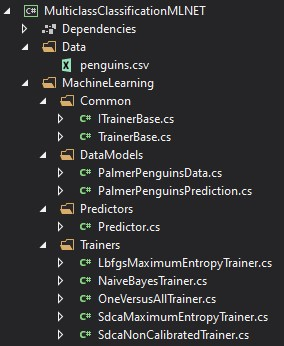Apart from the project structure, the architectural structure is the same as well:Again abstract TrainerBase class is running the show. Trainer algorithms implement this abstract class and define the algorithm from ML.NET. Model is saved and then loaded by the Predictor  which runs predictions on new samples.

### 5.2 Data Models

Data models are a bit more complex than the ones for binary classification. This is because we have more features now.

``````using Microsoft.ML.Data;

namespace MulticlassClassificationMLNET.MachineLearning.DataModels
{
/// <summary>
/// Models Palmer Penguins Binary Data.
/// </summary>
public class PalmerPenguinsData
{
public string Label { get; set; }

public string Island { get; set; }

public float CulmenLength { get; set; }

public float CulmenDepth { get; set; }

public float FliperLength { get; set; }

public float BodyMass { get; set; }

public string Sex { get; set; }
}

/// <summary>
/// Models Palmer Penguins Binary Prediction.
/// </summary>
public class PalmerPenguinsPrediction
{
[ColumnName("PredictedLabel")]
public string PredictedLabel { get; set; }
}
}``````

### 5.3 TrainerBase and ITrainerBase

The TrainerBase class is almost the same as in the previous example. In fact, it is just a little bit adjusted for the multiclass scenario. The biggest change is in the BuildDataProcessingPipeline() function. Here we applied a little bit of feature engineering. Namely, we converted features with string values into categorical features and coded the output and performed normalization:

``````using MulticlassClassificationMLNET.MachineLearning.DataModels;
using Microsoft.ML;
using Microsoft.ML.Calibrators;
using Microsoft.ML.Data;
using Microsoft.ML.Trainers;
using Microsoft.ML.Transforms;
using System;
using System.IO;

namespace MulticlassClassificationMLNET.MachineLearning.Common

{
/// <summary>
/// Base class for Trainers.
/// This class exposes methods for training, evaluating and saving ML Models.
/// Classes that inherit this class need to assing concrete model and name; and to implement data pre-processing.
/// </summary>
public abstract class TrainerBase<TParameters> : ITrainerBase
where TParameters : class
{
public string Name { get; protected set; }

protected static string ModelPath => Path.Combine(AppContext.BaseDirectory, "classification.mdl");

protected DataOperationsCatalog.TrainTestData _dataSplit;
protected ITrainerEstimator<MulticlassPredictionTransformer<TParameters>, TParameters> _model;
protected ITransformer _trainedModel;

protected TrainerBase()
{
MlContext = new MLContext(111);
}

/// <summary>
/// Train model on defined data.
/// </summary>
/// <param name="trainingFileName"></param>
public void Fit(string trainingFileName)
{
if (!File.Exists(trainingFileName))
{
throw new FileNotFoundException(\$"File {trainingFileName} doesn't exist.");
}

var dataProcessPipeline = BuildDataProcessingPipeline();
var trainingPipeline = dataProcessPipeline
.Append(_model)
.Append(MlContext.Transforms.Conversion.MapKeyToValue("PredictedLabel"));

_trainedModel = trainingPipeline.Fit(_dataSplit.TrainSet);
}

/// <summary>
/// Evaluate trained model.
/// </summary>
/// <returns>RegressionMetrics object which contain information about model performance.</returns>
public MulticlassClassificationMetrics Evaluate()
{
var testSetTransform = _trainedModel.Transform(_dataSplit.TestSet);

return MlContext.MulticlassClassification.Evaluate(testSetTransform);
}

/// <summary>
/// Save Model in the file.
/// </summary>
public void Save()
{
MlContext.Model.Save(_trainedModel, _dataSplit.TrainSet.Schema, ModelPath);
}

/// <summary>
/// Feature engeneering and data pre-processing.
/// </summary>
/// <returns>Data Processing Pipeline.</returns>
private EstimatorChain<NormalizingTransformer> BuildDataProcessingPipeline()
{
var dataProcessPipeline = MlContext.Transforms.Conversion.MapValueToKey(inputColumnName: nameof(PalmerPenguinsData.Label), outputColumnName: "Label")
.Append(MlContext.Transforms.Text.FeaturizeText(inputColumnName: "Sex", outputColumnName: "SexFeaturized"))
.Append(MlContext.Transforms.Text.FeaturizeText(inputColumnName: "Island", outputColumnName: "IslandFeaturized"))
.Append(MlContext.Transforms.Concatenate("Features",
"IslandFeaturized",
nameof(PalmerPenguinsData.CulmenLength),
nameof(PalmerPenguinsData.CulmenDepth),
nameof(PalmerPenguinsData.BodyMass),
nameof(PalmerPenguinsData.FliperLength),
"SexFeaturized"
))
.Append(MlContext.Transforms.NormalizeMinMax("Features", "Features"))
.AppendCacheCheckpoint(MlContext);

return dataProcessPipeline;
}

{
return MlContext.Data.TrainTestSplit(trainingDataView, testFraction: 0.3);
}
}
}
``````

### 5.4 Trainers

Trainers just use multiclass algorithms that we mentioned previously:

``````using Microsoft.ML;
using Microsoft.ML.Trainers;
using MulticlassClassificationMLNET.MachineLearning.Common;

namespace MulticlassClassificationMLNET.MachineLearning.Trainers
{
public class LbfgsMaximumEntropyTrainer : TrainerBase<MaximumEntropyModelParameters>
{
public LbfgsMaximumEntropyTrainer() : base()
{
Name = "LBFGS Maximum Entropy";
_model = MlContext.MulticlassClassification.Trainers
.LbfgsMaximumEntropy(labelColumnName: "Label", featureColumnName: "Features");
}
}

public class NaiveBayesTrainer : TrainerBase<NaiveBayesMulticlassModelParameters>
{
public NaiveBayesTrainer() : base()
{
Name = "Naive Bayes";
_model = MlContext.MulticlassClassification.Trainers
.NaiveBayes(labelColumnName: "Label", featureColumnName: "Features");
}
}

public class OneVersusAllTrainer : TrainerBase<OneVersusAllModelParameters>
{
public OneVersusAllTrainer() : base()
{
Name = "One Versus All";
_model = MlContext.MulticlassClassification.Trainers
.OneVersusAll(binaryEstimator: MlContext.BinaryClassification.Trainers.SgdCalibrated());
}
}

public class SdcaMaximumEntropyTrainer : TrainerBase<MaximumEntropyModelParameters>
{
public SdcaMaximumEntropyTrainer() : base()
{
Name = "Sdca Maximum Entropy";
_model = MlContext.MulticlassClassification.Trainers
.SdcaMaximumEntropy(labelColumnName: "Label", featureColumnName: "Features");
}
}

public class SdcaNonCalibratedTrainer : TrainerBase<LinearMulticlassModelParameters>
{
public SdcaNonCalibratedTrainer() : base()
{
Name = "Sdca NonCalibrated";
_model = MlContext.MulticlassClassification.Trainers
.SdcaNonCalibrated(labelColumnName: "Label", featureColumnName: "Features");
}
}
}
``````

### 5.5 Predictor

The Predictor is one of those class that looks completely the same:

``````using Microsoft.ML;
using MulticlassClassificationMLNET.MachineLearning.DataModels;
using System;
using System.IO;

namespace MulticlassClassificationMLNET.MachineLearning.Predictors
{
public class Predictor
{
protected static string ModelPath => Path.Combine(AppContext.BaseDirectory, "classification.mdl");

private ITransformer _model;

public Predictor()
{
_mlContext = new MLContext(111);
}

/// <summary>
/// Runs prediction on new data.
/// </summary>
/// <param name="newSample">New data sample.</param>
/// <returns>PalmerPenguinsData object, which contains predictions made by model.</returns>
public PalmerPenguinsPrediction Predict(PalmerPenguinsData newSample)
{

var predictionEngine = _mlContext.Model.CreatePredictionEngine<PalmerPenguinsData, PalmerPenguinsPrediction>(_model);

return predictionEngine.Predict(newSample);
}

{
if (!File.Exists(ModelPath))
{
throw new FileNotFoundException(\$"File {ModelPath} doesn't exist.");
}

{
}

if (_model == null)
{
throw new Exception(\$"Failed to load Model");
}
}
}
}``````

### 5.6 Usage and Results

Thanks to all the preparations, usage of this kind of system is rather simple:

``````using MulticlassClassificationMLNET.MachineLearning.Common;
using MulticlassClassificationMLNET.MachineLearning.DataModels;
using MulticlassClassificationMLNET.MachineLearning.Predictors;
using MulticlassClassificationMLNET.MachineLearning.Trainers;
using System;
using System.Collections.Generic;

namespace MulticlassClassificationMLNET
{
class Program
{
static void Main(string[] args)
{
var newSample = new PalmerPenguinsData
{
Island = "Torgersen",
CulmenDepth = 18.7f,
CulmenLength = 39.3f,
FliperLength = 180,
BodyMass = 3700,
Sex = "MALE"
};

var trainers = new List<ITrainerBase>
{
new LbfgsMaximumEntropyTrainer(),
new NaiveBayesTrainer(),
new OneVersusAllTrainer(),
new SdcaMaximumEntropyTrainer(),
new SdcaNonCalibratedTrainer()
};

trainers.ForEach(t => TrainEvaluatePredict(t, newSample));
}

static void TrainEvaluatePredict(ITrainerBase trainer, PalmerPenguinsData newSample)
{
Console.WriteLine("*******************************");
Console.WriteLine(\$"{ trainer.Name }");
Console.WriteLine("*******************************");

trainer.Fit("\\MulticlassClassificationMLNET\\Data\\penguins.csv");

var modelMetrics = trainer.Evaluate();

Console.WriteLine(\$"Macro Accuracy: {modelMetrics.MacroAccuracy:#.##}{Environment.NewLine}" +
\$"Micro Accuracy: {modelMetrics.MicroAccuracy:#.##}{Environment.NewLine}" +
\$"Log Loss: {modelMetrics.LogLoss:#.##}{Environment.NewLine}" +
\$"Log Loss Reduction: {modelMetrics.LogLossReduction:#.##}{Environment.NewLine}");

trainer.Save();

var predictor = new Predictor();
var prediction = predictor.Predict(newSample);
Console.WriteLine("------------------------------");
Console.WriteLine(\$"Prediction: {prediction.PredictedLabel:#.##}");
Console.WriteLine("------------------------------");
}
}
}
``````

In essence, we take the same approach as with the previous implementation. The TrainAndEvaluate() method receives an instance of the class that implements TrainerBase. Then we call Fit() and Evaluate() on this object to train and evaluate the model. Then we print out the metrics and save the model. Finally, we use the Predictor object to predict the penguin class of the new sample. In the Main() we create a list of multiclass trainers and call TrainAndEvaluate() for each object. The results are interesting:

``````*******************************
LBFGS Maximum Entropy
*******************************
Macro Accuracy: .96
Micro Accuracy: .95
Log Loss: .34
Log Loss Reduction: .67

------------------------------
------------------------------
*******************************
Naive Bayes
*******************************
Macro Accuracy: .77
Micro Accuracy: .68
Log Loss: 34.54
Log Loss Reduction: -32.75

------------------------------
------------------------------
*******************************
One Versus All
*******************************
Macro Accuracy: .76
Micro Accuracy: .67
Log Loss: .51
Log Loss Reduction: .51

------------------------------
------------------------------
*******************************
Sdca Maximum Entropy
*******************************
Macro Accuracy: .99
Micro Accuracy: .99
Log Loss: .08
Log Loss Reduction: .92

------------------------------
------------------------------
*******************************
Sdca NonCalibrated
*******************************
Macro Accuracy: .99
Micro Accuracy: .99
Log Loss: 28.08
Log Loss Reduction: -26.37

------------------------------
------------------------------``````

The SDCA algorithms had the best metrics and all algorithms gave the same answer.

## Conclusion

In this article, we covered three classification algorithms that are often used. We explored Logistic Regression, KNN and Naive Bayes. We had a chance to see how they function under the hood and use ML.NET for classification.#### Nikola M. Zivkovic

CAIO at Rubik's Code

Nikola M. Zivkovic a CAIO at Rubik’s Code and the author of book “Deep Learning for Programmers“. He is loves knowledge sharing, and he is experienced speaker. You can find him speaking at meetups, conferences and as a guest lecturer at the University of Novi Sad.

Rubik’s Code is a boutique data science and software service company with more than 10 years of experience in Machine Learning, Artificial Intelligence & Software development. Check out the services we provide.

Ultimate Guide to Machine Learning with Python

Everything from Python basics to the deployment of Machine Learning algorithms to production in one place.

Become a Machine Learning Superhero TODAY!